An Ecosystem of δ-Potentials - IVB Blip: Fermionic or Bosonic?   3rd September 2022 HOME "Simply put, quantum geometry is the appropriate modification of standard classical geometry to make it suitable for describing the physics of string theory." - Brian Greene, String Theory On Calabi-Yau Manifolds, arXiv:hep-th/9702155. "Finsler geometry is much broader than Riemannian geometry and can be treated in an elegant way.  It will be the subject of the basic course on differential geometry within the next ten years in many universities." - Shiing Shen Chern, Notices of the AMS, V0l.45(7), pp. 860-868, 1998. "Two unequal numbers being set out, and the less being continually subtracted in turn from the greater, if the number which is left never measures the one before it until an unit is left, the original numbers will be prime to one another." -Euclid's Elements, Book Seven, Proposition 1. "The principle of gaining knowledge of the external world from the behaviour of its infinitesimal parts is the mainspring of the theory of knowledge in infinitesimal physics as in Riemann’s geometry, and, indeed, the mainspring of all the eminent work of Riemann, in particular, that dealing with the theory of complex functions." - Hermann Weyl, Space-Time-Matter, Dover Publications, 1952. "The elevation of gauge fields to the level of the gravitational fields is a substantial achievement, but is by no means the end of the story. " - Lochlainn O'Raifeartaigh, The Dawning of Gauge Theory, Princeton Series in Physics, 1997.   *** ....continuing               The idea here, is that in a qualitative description we can express our rods and clocks system, in terms of the observers' relative efficiencies rather than their relative velocities.      We keep in mind that in the topological space (also the dB-space), the clock disappears.  And that in dB-space (deBroglie-Space), the rods' length and mass are correlated by jML.1          Let us define the relative efficiency for an observer in the reference frame- S', as η'.  In the case of an observer with relativistic K.E., η' = v2/c2.  The efficiency for Obsc defining dB-space is, ηc = 1.  We can rewrite the relativistic relationships for length, time, and mass as shown below: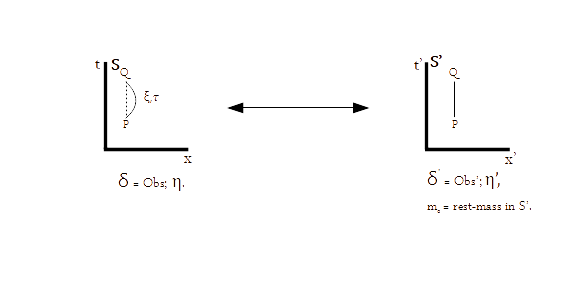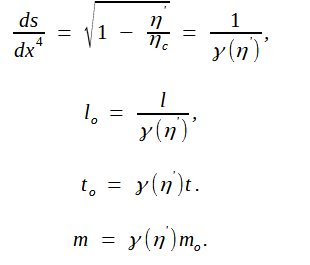We note that by describing these relationships in terms of the efficiency of the observer, we can also account for the case with very high structural potential energy.  Thus if an observer or a δ-function has a very rich information content, which translates into an equally high resource requirement (KE, PE, or KE + PE both), we can express the variation in arc-length ds with respect to an entropy dependent parameter t, represented as dx4, by the relationships just described above.       We do not connect the mass m in the above relationship to either inertial mass or gravitational mass unless we know for sure that the nature of δ-functions in S and S' are similar, i.e. Lorentz and Möbius invariance, are followed between S and S' frames. This is a rather important issue and we will discuss it a little later.       Let us now examine the observer's efficiency η in j-space. We have to decide on a criterion based on which the accuracy of the observer's measurements can be determined.  The upper limit for the Obsc efficiency ηc in q3-information space, is defined as 1j.    We note the following: In j-space, an observer is trying to determine the curve of the least disorder (CLD), which is formed by an infinite number of arcs2.  The arc of minimum radius or maximum curvature is determined by ηc. (It will not be a straight line.) A macroscopic observer ObsM (η << ηc) can not measure all the ∞j number of curves required to form CLD.  Please note that the curves here represent various paths (ds) in proper-time.  Again ∞j is determined by ηc.  Thus the criterion for determining η for a specific observer measuring a path PQ has the following requirements:     The Curvature of the path PQ if it is "zero-entropy measurement".   The number of path or curves or geodesics, required to be measured to complete path PQ .         We further note the following:   The finite physical dimension of a thin rod belonging to S'-frame (η'$\approx$1), will be measured as infinitesimal in S-frame.  Similarly a finite time interval measured by a clock in S'-frame (η'$\approx$1), will be measured as infinitely dilated in S-frame. The definition of the origin is determined by the observer being able to measure CLD.  Neither Obsc nor ObsM has this capability.  Therefore the region near the origin can not be measured in j-space.2  In j-space, the Obsc which has the maximum capacity in q3 (q = 3) information space, can not measure the origin and hence the probability of any observer belonging to q3-information space, being found at the origin of epoch-time⊗epoch-space (as determined by Obsi), is null.  In Schrödinger picture we can represent this situation in terms of the particle wave-functions and the probability densities as: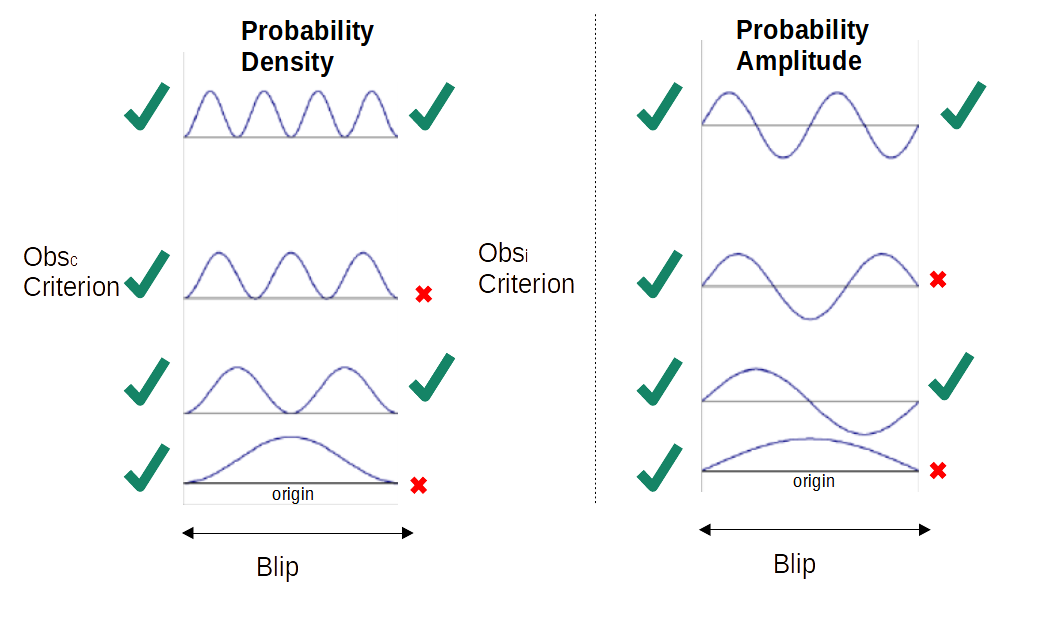The probability of a particle being found at the origin, the origin as measured by Obsi, is null and therefore in j-space we can have the energy states which correspond to anti-symmetric wave-functions or Fermions only.  (QCD)        However we can also have a measurement space in which the origin's definition is that based on the measurements corresponding to ηc.  In this case the probability of finding a particle at the origin is finite and we can have symmetric and anti-symmetric wave-functions both.  Hence in this case Fermions and Bosons are both exist in various energy states including the ground state GSj. (String Theory)   It is clear that the measurement of the true origin is not possible even in dB-space, which represent the topological space underlying various manifolds in q3 information space.  In essence, the measurement of the true origin requires capabilities not possible in Newtonian frame of reference, nor in dB space.  Therefore the structures existing in Newtonian-Space, Schrödinger-Space or dB-space, will measure CLD as a quantum problem.  In other words, every possible structure in Blip will measure CLD as a quantum problem.  (This is the definition of the unification in j-space.)  We can also infer that the discreteness is an intrinsic property of Blip. More importantly we can make the statement that mass must exist in Blip.   The argument goes like this, in the potential well or the harmonic oscillator problem, a particle in an excited  state can transition to a lower energy state by emitting a photon with a positive momentum.  Similarly a particle can move up to a higher energy state by absorbing a photon.  The particle in a higher energy state therefore has higher mass  than the same particle in a lower energy state.  We can not determine that whether a particle in the ground state should also have a mass which is either finite or zero.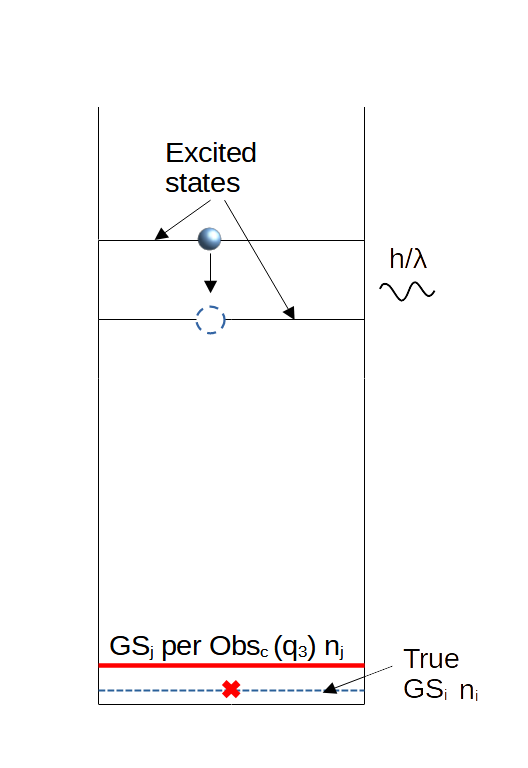However what we can say is that a particle in an excited state would have a finite mass. In measurement space, we just showed that the origin as determined by Obsi (true-origin, Oi), can not precisely determined by the resources available  in q3-information space or q3-universe.  Subsequently the probability of finding an particle at the origin has to be zero.  Moving over to the harmonic oscillator description, we can state that, the probability of finding a particle in the energy states corresponding to n = 0i, 2i, 4i, ... (2ni), is null.  If the particle, in this case Blip (the universe in q3-information space), can not exist in the Ground State corresponding to true-origin, also called Zero-point energy state or GSi, therefore Blip must exist in one of the excited states corresponding to n = 1i, 3i, 5i....(2i⊗ni $⨁$ 1i).  And since Blip is in one of the excited states, then it must have a finite mass.  Please note that we do not know what the quanta is in this case.  It can be a Higgs-boson or something even more profound. The important inference here, is that mass must exist in a measurement space.  Also that the allowed energy levels for particles are not continuous, instead they are discrete.  It does not matter whether the particles are represented by either a singular delta function or a composite delta function or whether they are either microscopic or macroscopic structures, in Blip.     Let us wind down for now, by summarizing our discussions so far:      We have described the length-contraction and time-dilation in terms of observers' relative capabilities (η's).  The consequence is that the higher information spaces are measured as infinitesimally small and frozen with respect to the time scale of S-frame.  The reason being that observer in S-frame is measuring the information from S'-frame, and consequences are proper-space and proper-time geodesics.  While measuring a very high information content structure or δ-potential, the progress of the geodesic in S-frame is infinitesimal for the measurements based on the S-Observer's Hamiltonian (geodesics is almost perpendicular to the actual time axis t of S-Observer).  Thus an observer in S-frame, will always be in the state of measurement for Case-2 and Case-3. Since SLE is a structure consisting of infinite number of arcs with the curvature varying from 0j to ∞j, the geodesic corresponding to SLE-measurement is virtually non-existent or infinitesimal in S-frame.  Blip the universe belonging to AKU the amoeba, exists in S-frame.  Hence every possible structure, microscopic or macroscopic, belonging to Blip will be in the state of measurement for the duration of the epoch.  And since Blip is equivalent to q3-information space, every structure in Blip is in the state of quantum measurement of SLE.  Which means that energy levels available in Blip, for structure formation are discrete.  Therefore the macroscopic and microscopic structures will have discrete distributions across q3-continuum.  We already know that these structures will exists in 3-D.  (We are yet to discuss though, how or why various structures or different types of $\delta$-potentials are distributed with respect to each other in j-space.) The criterion for measurement of the origin or 0i is provided by Obsi.  Therefore the origin cannot be measured by Obsc or ObsM with their respective Hamiltonian, unless additional resources or information is provided.  It means that probability of finding a q3-observer in GSi is null.  The lowest energy state a Blip observer can exist in, is the energy state corresponding to ni = 1i.3  The wavefunction for ni = 1i state, is anti-symmetric.  The measurement space for a q3-observer is Fermionic and hence so is Blip. Since the Blip is always in an energy state other than GSi, Blip and all the structures within, must have finite mass.   We can also have a description of a lower information content space, based on the origin 0j per measurements by Obsc (instead of the origin Oi defined by Obsi). In this case the probability of  finding a particle in GSj, is finite.  Bosons and Fermions both can exist.  However GSj itself is equivalent to an excited state, corresponding to the harmonic oscillator with GSi as its ground state.  Therefore a q3-universe and the objects within, will always have finite mass. Since we are measuring an infinite information source, which means that the entropy in the measurements is increasing continuously.  Which implies that we are moving away from GSi (GSj as well), with the progression of time.  Therefore the particle or Blip is getting heavier and heavier.  This mass is the "inertial mass" and it is measured in the region-I of Kruskal-Szekeres coordinates.  Increasing entropy also means that that the event-horizon of the black-hole towards which Blip is progressing, is expanding and by the time the time Blip-epoch is over, Blip will be firmly entrenched in a Black-hole unless the information Eco-system is changed i.e. the value of the fine-structure constant $\alpha$ is increased. The "inertial mass" exists because the observer cannot measure or does not have the precise information about GSi.4  We can briefly explore the symmetry breaking in the measurement space, as an observer moves from GSj to GSi by strengthening its Hamiltonian from Hj to Hi.  We know that j-space is anti-symmetric in nature per Obsi, however ObsM will measure it as a regular Schrödinger-space where both anti-symmetric and symmetric wave-functions are possible.  Therefore we can also say that symmetry in j-space allows anti-symmetric and symmetric wave-functions,  And by breaking a symmetry the observer moves into the Fermionic space.   Equivalently a macroscopic observer ObsM measures a Quartic-Potential as a Quadratic-Potential due to lack of resources, as shown below.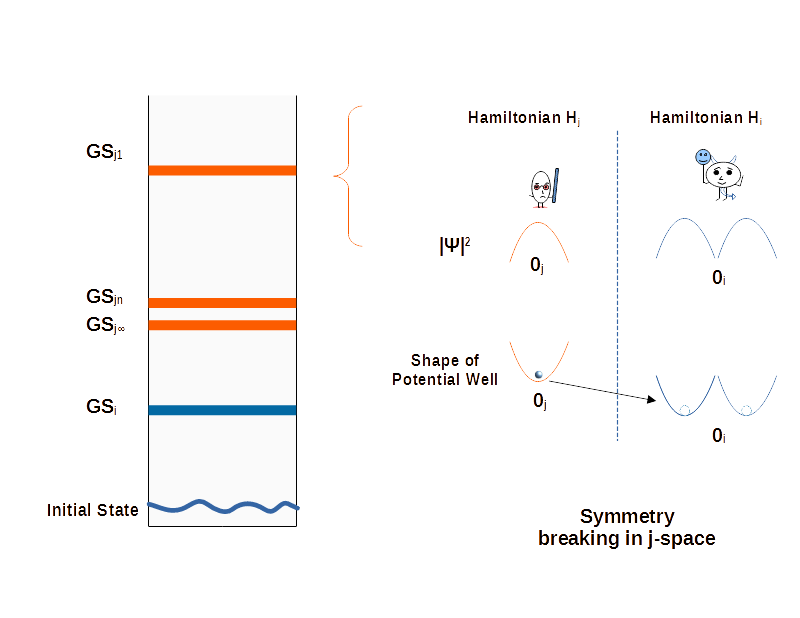Here saying that the particle has moved into a lower energy state is slightly misleading.  Lower energy states especially those close to GSi, are extremely powerful.  We simply do not have resources in q3-information space to break VT-symmetries, to achieve these states.  We will talk about this issue some other time.        We will be discussing some of the following ideas in forthcoming blogs:   The structures lasting the duration on an epoch can not exist in the description based on Bosons only.  The essential nature of the discrete measurement space, is Fermionic with a Boson representing the quanta of interaction between the scalar field formed by j-pixels and a Fermion.  The Fermionic nature of j-space opens up the possibility that Kaluza-Klein theory, which unified EM and Gravitational theory, is correct.  We need to understand this theory and revisit Klein's explanation. Due to the Fermionic nature of the j-space, the structure formation is based on non-abelian theories such as Yang-Mills (Pauli).  The concepts used in QCD become very important in j-space.  The microscopic and macroscopic structures in Blip, must follow Yang-Mills(Pauli) theory. We keep in mind that these results originate from the fact that given an arbitrary surface in the measurement space, if we keep contracting it by more and more precise measurements, it will not contract to a singular point but to the surface of least energy (SLE).  The objective is to make our measurements more and more precise.  Cosmology is a tool worth investment, as the machinery defining inner mechanisms is already there.  The structures in nature may be colossal, they are still in the quantum state of measurement.  We just need to understand the information being provided by these structures as our own Hamiltonian becomes more and more sophisticated, (Genetic evolution × Knowledge × Resources). If the j-space as measured by Obsc is Fermionic in nature, then the best cosmological tool available to a q3-observer is a white dwarf.  The ultimate tool for measurements of-course, is a Black-hole in which every single bit of information is completely unique to each other.  It will be very interesting to measure such structure with limited resources available in q3-universe. It is also very clear that the complete physical description can not be based on a collection of points alone, and we need a theory of curves combined with an ensemble of $\delta$-potentials.  Perhaps Finsler geometry along with the redefinition of the parallel-transport, can provide a better understanding.    So how do we think of δ-potential(s) in the measurement-system we are discussing?  Please note that we already know that the δ-potential from a higher information space will show up as a coherent state in Blip.  Our immediate objective is to understand the structures in the universe as we know it, represented by q = 3 information state.   to be continued..............    ____________________ 1. The Lorentz factor , cancels out for the product between m and l relationships in S-frame i.e. m x l = mo x l'. 2.  The origin in j-space corresponding to q = 3 can never be precisely determined, which we already know, since we do not have an observer who can measure SLC in q = 3 information space.  Therefore the "Well" here, can have the dimension of the universe and it will still be a quantum problem of measurement.  3.  Although we are using the analogy with an Harmonic Oscillator to simplify our arguments, the Harmonic Oscillator and Ladder Operators are not valid description tools for the curved space-time.  They are useful only in the flat space-time. 4.  We also have Zero-Entropy mass mZE in j-space.   *** Previous Blogs:   EPR Paradox-I  Nutshell-2015Information on www.ijspace.org is licensed under a Creative Commons Attribution 4.0 International License. Attribution — You must give appropriate credit, provide a link to the license, and indicate if changes were made. You may do so in any reasonable manner, but not in any way that suggests the licensor endorses you or your use. No additional restrictions — You may not apply legal terms or technological measures that legally restrict others from doing anything the license permits. This is a human-readable summary of (and not a substitute for) the license.